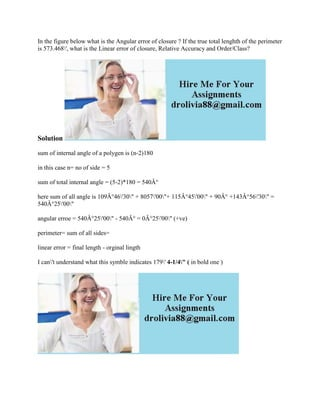Se está descargando tu SlideShare. ×

# In the figure below what is the Angular error of closure - If the tru.docx

Anuncio
Anuncio
Anuncio
Anuncio
Anuncio
Anuncio
Anuncio
Anuncio
Anuncio
Anuncio
AnuncioCargando en…3
×

1 de 1 Anuncio

# In the figure below what is the Angular error of closure - If the tru.docx

In the figure below what is the Angular error of closure ? If the true total lenghth of the perimeter is 573.468\', what is the Linear error of closure, Relative Accuracy and Order/Class?
Solution
sum of internal angle of a polygen is (n-2)180
in this case n= no of side = 5
sum of total internal angle = (5-2)*180 = 540Â°
here sum of all angle is 109Â°46\'30\" + 8057\'00\"+ 115Â°45\'00\" + 90Â° +143Â°56\'30\" = 540Â°25\'00\"
angular erroe = 540Â°25\'00\" - 540Â° = 0Â°25\'00\" (+ve)
perimeter= sum of all sides=
linear error = final length - orginal lingth
I can\'t understand what this symble indicates 179\' 4-1/4\" ( in bold one )
.

In the figure below what is the Angular error of closure ? If the true total lenghth of the perimeter is 573.468\', what is the Linear error of closure, Relative Accuracy and Order/Class?
Solution
sum of internal angle of a polygen is (n-2)180
in this case n= no of side = 5
sum of total internal angle = (5-2)*180 = 540Â°
here sum of all angle is 109Â°46\'30\" + 8057\'00\"+ 115Â°45\'00\" + 90Â° +143Â°56\'30\" = 540Â°25\'00\"
angular erroe = 540Â°25\'00\" - 540Â° = 0Â°25\'00\" (+ve)
perimeter= sum of all sides=
linear error = final length - orginal lingth
I can\'t understand what this symble indicates 179\' 4-1/4\" ( in bold one )
.

Anuncio
Anuncio

### In the figure below what is the Angular error of closure - If the tru.docx

1. 1. In the figure below what is the Angular error of closure ? If the true total lenghth of the perimeter is 573.468', what is the Linear error of closure, Relative Accuracy and Order/Class? Solution sum of internal angle of a polygen is (n-2)180 in this case n= no of side = 5 sum of total internal angle = (5-2)*180 = 540Â° here sum of all angle is 109Â°46'30" + 8057'00"+ 115Â°45'00" + 90Â° +143Â°56'30" = 540Â°25'00" angular erroe = 540Â°25'00" - 540Â° = 0Â°25'00" (+ve) perimeter= sum of all sides= linear error = final length - orginal lingth I can't understand what this symble indicates 179' 4-1/4" ( in bold one )